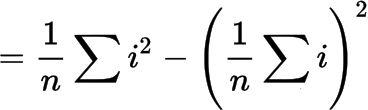# What are the advantages/disadvantages of uLisp vs C/C++?

#1

A visitor to my electronics blog, Technoblogy, recently asked this question, and I thought it would make a useful topic for discussion on this forum.

To make the question more targeted I’m going to assume we mean:

What are the advantages/disadvantages of uLisp vs C/C++ for developing applications on microcontroller boards for embedded applications?

Here’s my initial list of pros and cons:

### Advantages of uLisp

• Easier to prototype ideas. For example, you can use functional programming to solve problems that are harder to express using imperative programming.

• Higher-level constructs mean that you can often achieve the same result with a shorter, simpler program.

• String and list handling makes some types of problem easier to solve.

• No need to recompile between changes to the program, making development quicker. For example, you can type a digitalwrite command in the Serial Monitor and see an LED flash immediately.

### Disadvantages of uLisp

• Slower, because it’s an interpreter, so applications requiring microsecond timing aren’t practical.

• There’s a memory overhead due to the uLisp interpreter.

#2

By the way, this is another post relevant to this question:

#3

I’ve been asked to expand on this point:

Easier to prototype ideas. For example, you can use functional programming to solve problems that are harder to express using imperative programming.

Lisp allows you to write in either a functional or imperative style, or a mixture of the two.

As an example of what functional and imperative mean, here’s the same problem approached in an imperative and functional style.

Suppose we want to calculate the variance of a set of data values:

1 4 9 16 25 36 49

We can use the equation:### Imperative

Here’s the imperative approach. For convenience we first define a sq function:

``````(defun sq (x) (* x x))
``````

The imperative version is like a recipe. We set up the three variables n, sum, and sumsq, and then step through the data a value at a time, updating the three variables:

``````(defun variance-i (data)
(let ((n (length data))
(sum 0)
(sumsq 0)
d)
(dotimes (i n)
(setq d (nth i data))
(setq sum (+ sum d))
(setq sumsq (+ sumsq (sq d))))
(- (/ sumsq n) (sq (/ sum n)))))
``````

This is almost exactly how you would express the program in an imperative language such as C or C++:

``````const int ndata = 7;
float dataset[ndata] = {1, 4, 9, 16, 25, 36, 49 };

float variance (float* data, int n) {
int sum = 0, sumsq = 0;
for (int i=0; i<ndata; i++) {
float d = data[i];
sum = sum + d;
sumsq = sumsq + sq(d);
}
return sumsq/n - sq(sum/n);
}
``````

Let’s check that it works:

``````> (variance-i '(1 4 9 16 25 36 49))
268
``````

### Functional

Now the functional approach. This approach consists of building up a series of functions that produce each of the intermediate results that we need. The functions n and sum each take a list of numbers, and return the number of values in the list, and the sum of those values, respectively:

``````(defun n (data) (length data))
(defun sum (data) (apply + data))
``````

The function squares takes a list of numbers and returns a list containing the square of each number:

``````(defun squares (data) (mapcar sq data))
``````

Now we are in a position to write the function variance :

``````(defun variance-f (data)
(- (/ (sum (squares data)) (n data))
(sq (/ (sum data) (n data)))))
``````

Note that the function variance-f is a direct expression of the definition of variance. We haven’t had to construct a recipe to calculate the variance.

Again, testing the function:

``````> (variance-f '(1 4 9 16 25 36 49))
268``````